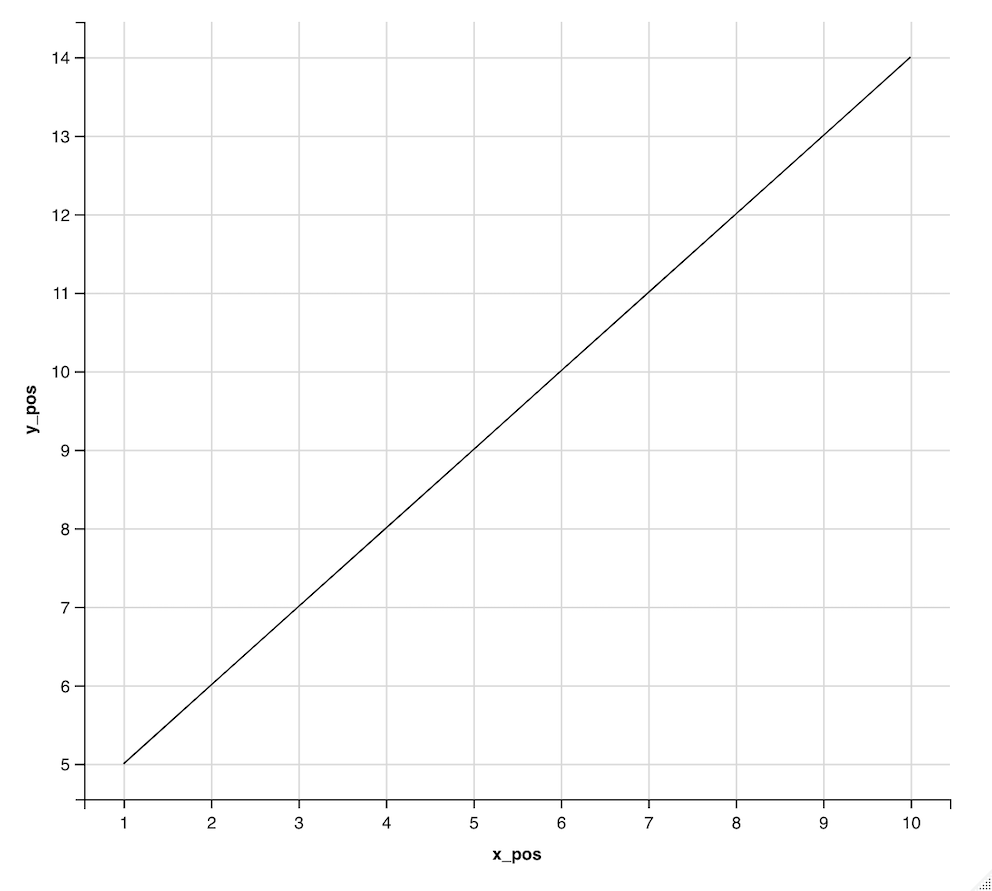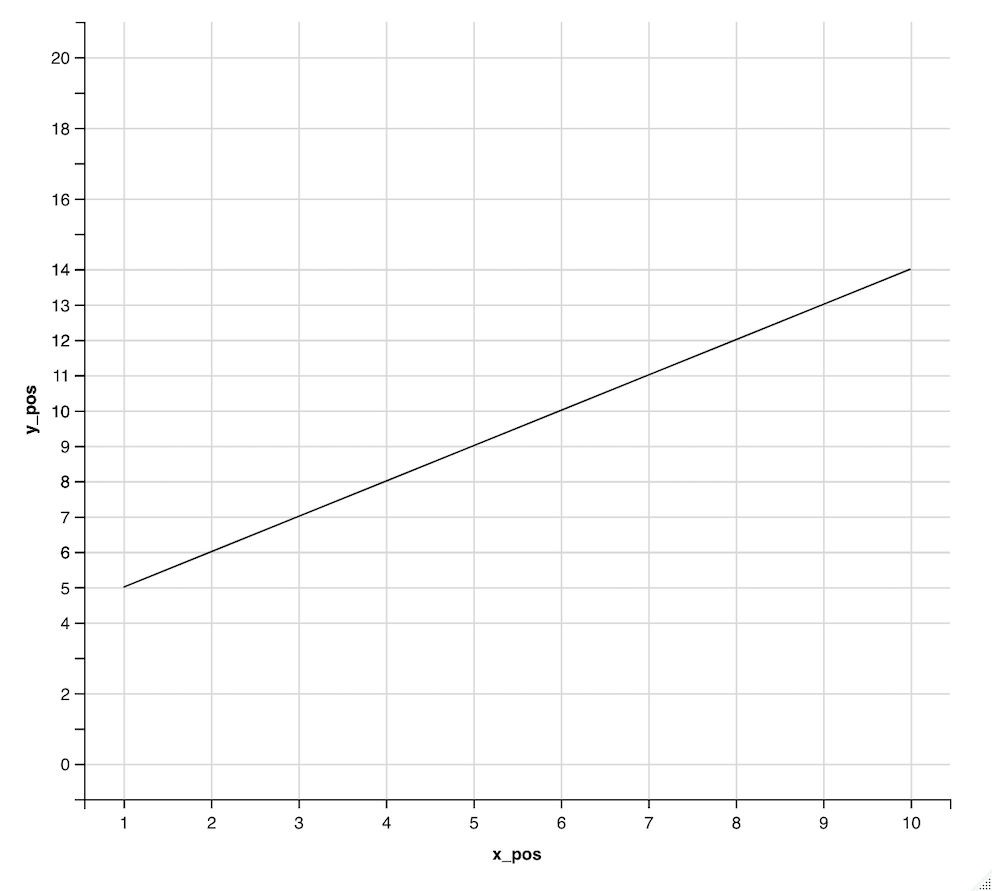GFG App
Open AppBrowser
Continue

# How to change maximum and minimum label in ggvis plot in R

A plot in R is used to depict the data in a pictorial form, representing the points using the coordinates. A plot has two axes, namely, the x and y axes, respectively. The x and y axes are represented using the labels, the minimum and maximum, respectively. There are multiple external packages in R which are used to draw plots.

The ggplot2 library in R is used to make a graphical representation of the data provided. The package can be downloaded and installed into the working space using the following command :

`install.packages("ggplot2")`

A data frame is initially created using the data.frame() method. The data points are constructed in the data frame. The data frame is subjected to the ggvis operations using the pipe operator. The ggvis method is used to start ggvis graphical window. The ggvis method has the following syntax :

ggvis( data , mp1, mp2.,)

Arguments :

• data – The dataset to plot
• mp1, mp2,.. – The map variables to plot

The add_axis method is then added to derive the labeling for the axes of the plotted graph. It can be used to override the default value for the axes.

add_axis( vis, axes , values = )

Arguments :

• vis – The ggvis object to use for plotting
• axes – The axes to be used for plotting
• values – The values to be used for deriving the tick marks for the label

The labels are by default based on the values of the x and y data points, respectively. For instance, in the graph shown below, the y-axis labels are designated from the values ranging from 5 to 14. The data points in the x_pos are between the interval 1 to 10, whereas the data points in the y_pos are in the interval 5 to 14, therefore, the labels are plotted between these values.

## R

 `# installing the required libraries  ` `library``(ggplot2) ` `library``(ggvis) ` ` `  `# creating the data frame by defining the ` `# x and y coordinates respectively ` `x_pos <- 1:10 ` ` `  `# defining the y axis  ` `y_pos = 5:14 ` ` `  `# creating the data frame ` `data_frame = ``data.frame``(x_pos, y_pos ) ` `print``(``"Data Frame"``) ` `print``(data_frame) ` ` `  `# plotting the tick marks on the axes ` `data_frame %>% ` `  ``ggvis``(~x_pos,~y_pos) %>% ` `  ``layer_lines``() %>% ` `  ``# adding customised tick marks to the axes ` `  ``add_axis``(``"x"``, values=x_pos)  %>% ` `  ``add_axis``(``"y"``, values = y_pos)`

Output

``` "Data Frame"
x_pos y_pos
1      1     5
2      2     6
3      3     7
4      4     8
5      5     9
6      6    10
7      7    11
8      8    12
9      9    13
10    10    14```The scale_numeric() method in the ggvis package is used to create the labeling of the y axis. It is used to provide the custom labels of the y axis.

scale_numeric ( axis, domain = )

Arguments :

• Axis – The axis to be used for plotting the custom scale of the y axis
• domain – The range to specify the upper and lower bound of the specified axis, respectively.

The following code snippet changes the domain of the y coordinates to the values in the interval (0,20). The values are plotted beginning from the value equivalent to 5 until 14.

## R

 `# installing the required libraries  ` `library``(ggplot2) ` `library``(ggvis) ` ` `  `# creating the data frame by defining  ` `# the x and y coordinates respectively ` `x_pos <- 1:10 ` ` `  `# defining the y axis  ` `y_pos = 5:14 ` ` `  `# creating the data frame ` `data_frame = ``data.frame``(x_pos, y_pos ) ` `print``(``"Data Frame"``) ` `print``(data_frame) ` ` `  `# plotting the tick marks on the axes ` `data_frame %>% ` `  ``ggvis``(~x_pos,~y_pos) %>% ` `  ``layer_lines``() %>% ` ` `  `  ``# adding customised tick marks to the axes ` `  ``add_axis``(``"x"``, values=x_pos)  %>% ` `  ``add_axis``(``"y"``, values = y_pos) %>% ` ` `  `  ``# specifying the range of y labels ` `  ``scale_numeric``(``"y"``, domain = ``c``(0, 20)) %>% ` `   `  `  ``# dividing the axis in the ratio of 1 segment ` `  ``add_axis``(``"y"``, subdivide = 1)`

Output

``` "Data Frame"
x_pos y_pos
1      1     5
2      2     6
3      3     7
4      4     8
5      5     9
6      6    10
7      7    11
8      8    12
9      9    13
10    10    14```My Personal Notes arrow_drop_up